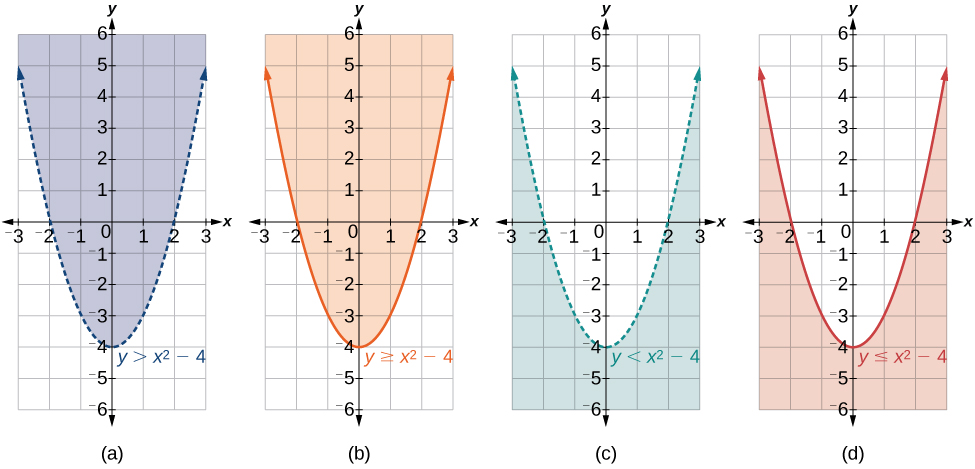# 11.3 Systems of nonlinear equations and inequalities: two variables  (Page 3/9)

 Page 3 / 9

## Solving a system of nonlinear equations representing a circle and an ellipse

Solve the system of nonlinear equations.

$\begin{array}{rr}\hfill {x}^{2}+{y}^{2}=26\text{\hspace{0.17em}}\text{\hspace{0.17em}}\text{\hspace{0.17em}}& \hfill \left(1\right)\\ \hfill 3{x}^{2}+25{y}^{2}=100& \hfill \left(2\right)\end{array}$

Let’s begin by multiplying equation (1) by $\text{\hspace{0.17em}}-3,\text{}$ and adding it to equation (2).

After we add the two equations together, we solve for $\text{\hspace{0.17em}}y.$

$\begin{array}{l}{y}^{2}=1\hfill \\ \text{\hspace{0.17em}}\text{\hspace{0.17em}}\text{\hspace{0.17em}}y=±\sqrt{1}=±1\hfill \end{array}$

Substitute $\text{\hspace{0.17em}}y=±1\text{\hspace{0.17em}}$ into one of the equations and solve for $\text{\hspace{0.17em}}x.$

There are four solutions: $\text{\hspace{0.17em}}\left(5,1\right),\left(-5,1\right),\left(5,-1\right),\text{and}\text{\hspace{0.17em}}\left(-5,-1\right).\text{\hspace{0.17em}}$ See [link] .

Find the solution set for the given system of nonlinear equations.

$\begin{array}{c}4{x}^{2}+{y}^{2}=13\\ \text{\hspace{0.17em}}\text{\hspace{0.17em}}\text{\hspace{0.17em}}{x}^{2}+{y}^{2}=10\end{array}$

$\left\{\left(1,3\right),\left(1,-3\right),\left(-1,3\right),\left(-1,-3\right)\right\}$

## Graphing a nonlinear inequality

All of the equations in the systems that we have encountered so far have involved equalities, but we may also encounter systems that involve inequalities. We have already learned to graph linear inequalities by graphing the corresponding equation, and then shading the region represented by the inequality symbol. Now, we will follow similar steps to graph a nonlinear inequality so that we can learn to solve systems of nonlinear inequalities. A nonlinear inequality    is an inequality containing a nonlinear expression. Graphing a nonlinear inequality is much like graphing a linear inequality.

Recall that when the inequality is greater than, $\text{\hspace{0.17em}}y>a,$ or less than, $\text{\hspace{0.17em}}y the graph is drawn with a dashed line. When the inequality is greater than or equal to, $\text{\hspace{0.17em}}y\ge a,\text{}$ or less than or equal to, $\text{\hspace{0.17em}}y\le a,\text{}$ the graph is drawn with a solid line. The graphs will create regions in the plane, and we will test each region for a solution. If one point in the region works, the whole region works. That is the region we shade. See [link] .(a) an example of   y > a ;   (b) an example of   y ≥ a ;   (c) an example of   y < a ;   (d) an example of   y ≤ a

Given an inequality bounded by a parabola, sketch a graph.

1. Graph the parabola as if it were an equation. This is the boundary for the region that is the solution set.
2. If the boundary is included in the region (the operator is $\text{\hspace{0.17em}}\le \text{\hspace{0.17em}}$ or $\text{\hspace{0.17em}}\ge$ ), the parabola is graphed as a solid line.
3. If the boundary is not included in the region (the operator is<or>), the parabola is graphed as a dashed line.
4. Test a point in one of the regions to determine whether it satisfies the inequality statement. If the statement is true, the solution set is the region including the point. If the statement is false, the solution set is the region on the other side of the boundary line.
5. Shade the region representing the solution set.

## Graphing an inequality for a parabola

Graph the inequality $\text{\hspace{0.17em}}y>{x}^{2}+1.$

First, graph the corresponding equation $\text{\hspace{0.17em}}y={x}^{2}+1.\text{\hspace{0.17em}}$ Since $\text{\hspace{0.17em}}y>{x}^{2}+1\text{\hspace{0.17em}}$ has a greater than symbol, we draw the graph with a dashed line. Then we choose points to test both inside and outside the parabola. Let’s test the points
$\left(0,2\right)\text{\hspace{0.17em}}$ and $\text{\hspace{0.17em}}\left(2,0\right).\text{\hspace{0.17em}}$ One point is clearly inside the parabola and the other point is clearly outside.

$\begin{array}{ll}y>{x}^{2}+1\hfill & \hfill \\ 2>{\left(0\right)}^{2}+1\hfill & \hfill \\ 2>1\hfill & \text{True}\hfill \\ \hfill & \hfill \\ \hfill & \hfill \\ \hfill & \hfill \\ 0>{\left(2\right)}^{2}+1\hfill & \hfill \\ 0>5\hfill & \text{False}\hfill \end{array}$

The graph is shown in [link] . We can see that the solution set consists of all points inside the parabola, but not on the graph itself.

stock therom F=(x2+y2) i-2xy J jaha x=a y=o y=b
root under 3-root under 2 by 5 y square
The sum of the first n terms of a certain series is 2^n-1, Show that , this series is Geometric and Find the formula of the n^th
cosA\1+sinA=secA-tanA
why two x + seven is equal to nineteen.
The numbers cannot be combined with the x
Othman
2x + 7 =19
humberto
2x +7=19. 2x=19 - 7 2x=12 x=6
Yvonne
because x is 6
SAIDI
what is the best practice that will address the issue on this topic? anyone who can help me. i'm working on my action research.
simplify each radical by removing as many factors as possible (a) √75
how is infinity bidder from undefined?
what is the value of x in 4x-2+3
give the complete question
Shanky
4x=3-2 4x=1 x=1+4 x=5 5x
Olaiya
hi can you give another equation I'd like to solve it
Daniel
what is the value of x in 4x-2+3
Olaiya
if 4x-2+3 = 0 then 4x = 2-3 4x = -1 x = -(1÷4) is the answer.
Jacob
4x-2+3 4x=-3+2 4×=-1 4×/4=-1/4
LUTHO
then x=-1/4
LUTHO
4x-2+3 4x=-3+2 4x=-1 4x÷4=-1÷4 x=-1÷4
LUTHO
A research student is working with a culture of bacteria that doubles in size every twenty minutes. The initial population count was  1350  bacteria. Rounding to five significant digits, write an exponential equation representing this situation. To the nearest whole number, what is the population size after  3  hours?
v=lbh calculate the volume if i.l=5cm, b=2cm ,h=3cm
Need help with math
Peya
can you help me on this topic of Geometry if l help you
litshani
( cosec Q _ cot Q ) whole spuare = 1_cosQ / 1+cosQ
A guy wire for a suspension bridge runs from the ground diagonally to the top of the closest pylon to make a triangle. We can use the Pythagorean Theorem to find the length of guy wire needed. The square of the distance between the wire on the ground and the pylon on the ground is 90,000 feet. The square of the height of the pylon is 160,000 feet. So, the length of the guy wire can be found by evaluating √(90000+160000). What is the length of the guy wire?
the indicated sum of a sequence is known as
how do I attempted a trig number as a starterByByBy Edgar DelgadoBy OpenStaxBy OpenStaxBy Maureen MillerBy OpenStaxBy Marion CabalfinBy OpenStaxBy OpenStaxBy Sandy YamaneBy Robert Murphy